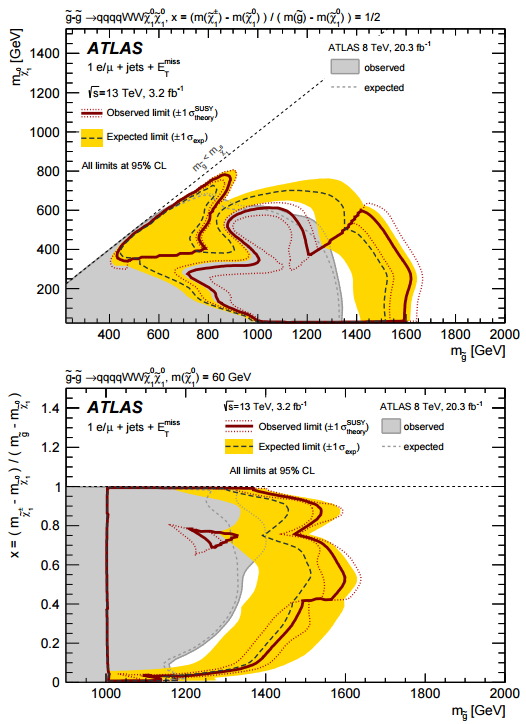Monday, May 16, 2016

ATLAS: an amusing 2.1-sigma gluino-muon-multijet island excess

ATLAS released an interesting preprint
Search for gluinos in events with an isolated lepton, jets and missing transverse momentum at $$\sqrt{s} = 13\TeV$$ with the ATLAS detector
in which gluino pairs were searched in final states with MET, many jets, and a single lepton. There were six signal regions. The last, sixth one, showed a mild but interesting excess. $$2.5\pm 0.7$$ events were expected with a muon (thanks, Bill), but eight events were observed.

The excess looks intriguing when it's visualized on Figure 6.

The plot has two parts:The observed thick red exclusion line is generally similar to the dashed black expected exclusion line. But on the upper picture, there is a clear "downward tooth" of the red line around the gluino mass of $$1200-1300\GeV$$ and the lightest neutralino mass around $$400-600\GeV$$, potentially properties of particles that may exist according to this hint.

On the second diagram, the excess looks like an island with $$m_{\tilde g} \sim 1250\GeV$$ and the ratio of two mass differences (lightest chargino minus first lightest neutralino OVER gluino minus lightest neutralino) equal to $$0.75$$ or so. However, the plot isn't quite showing the neighborhood of the most interesting values indicated by the upper plot because in the lower plot, the lightest neutralino is assumed to weigh $$60\GeV$$.

The island-like shape of the exclusion line on the lower picture is interesting, nevertheless. Note that this is what the exclusion lines look like when all the wrong values of the mass are excluded and the correct mass is discovered. In this sense, the lower picture could already be a sketch of a discovery paper.

At any rate, if you go through the LHC category or search for a gluino on this blog, I think you will agree that it's far from the first hint of a gluino close enough to $$1200\GeV$$ and a lightest neutralino in the $$600\GeV$$ category. I am extremely far from any form of certainty that the gluino has to be found near these masses but if you offer me 100-to-1 odds like Jester did, I will happily make the bet again (or increase the existing one, if you wish).